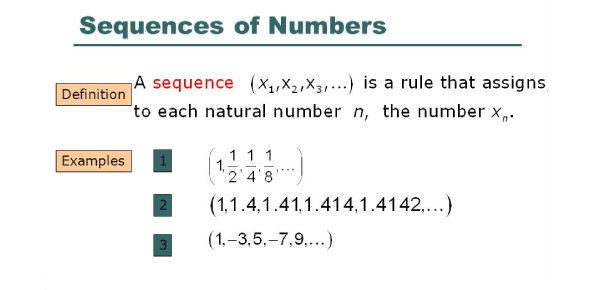# Maths Quiz- Unit 2. Sequences And Series Of Real Numbers

20 Questions | Total Attempts: 2730SettingsAn arithmetic sequence of numbers is such that the difference between two progressive numbers is the same or follows a particular pattern. Having covered some of the mathematics problems on this it is now time for a quick quiz. Give it a try and you are only allowed twenty minutes.

• 1.
which one of the  following  is not true
• A.

Every function represents a sequence

• B.

A sequence `is a real valued function defined on N

• C.

A sequence may have infinitely many terms

• D.

A sequence may have a finite number of terms

• 2.
The  8 th  term  of the  sequence  1,1,2,3,5,8,,..........is
• A.

21

• B.

25

• C.

24

• D.

23

• 3.
• A.

1/30

• B.

1/24

• C.

1/22

• D.

1/18

• 4.
If  a , b,c   are   in AP   then       is equal  to
• A.

1

• B.

A/c

• C.

B/c

• D.

A/b

• 5.
If  a,b,c,l,m    are  in AP   then the value of    a-4b+6c-4l+m  is
• A.

0

• B.

1

• C.

2

• D.

3

• 6.
• A.

an AP

• B.

A GP

• C.

A constant sequence

• D.

Neither AP nor GP

• 7.
then the  13 th term of the  AP is     (1)     0    (2)  3/2     (3)  12   (4)  14
• A.

(1)

• B.

(4)

• C.

(3)

• D.

(2)

• 8.
is
• A.

An AP

• B.

A GP

• C.

Neither AP nor GP

• D.

A constant sequence

• 9.
If  k+2 , 4k-6 , 3k-2 are  the three consecutive terms   of an AP  , then the  value of k    is
• A.

3

• B.

2

• C.

5

• D.

4

• 10.
If  a,,b,c, l,m,n , are  in AP ., then   3a+7 , 3b+7 , 3c+7 , 3l+7 , 3m+7 , 3n+7     form
• A.

an AP

• B.

A GP

• C.

A constant sequence

• D.

Neither AP nor GP

• 11.
If the   third term of a G.P  is 2 , then the  product  of first 5 terms  is   (1)      (2)  10  (3)    (4) 15
• A.

(1)

• B.

(3)

• C.

(2)

• D.

(4)

• 12.
If  a , b , c   are  in    G.P  then     is equal to
• A.

A/b

• B.

B/a

• C.

A/c

• D.

C/b

• 13.
If  x , 2x+2  , 3x+3 ,   are in G.P  then     5x , 10x+10  , 15x+15     form
• A.

a G.P

• B.

An AP

• C.

A constant sequence

• D.

Neither A.P nor G.P

• 14.
The  sequence  -3  , -3 , -3  , .......   is
• A.

Both A.P and G.P

• B.

An A.P only

• C.

A G.P only

• D.

Neither A.P nor G.P

• 15.
• A.

16

• B.

1/16

• C.

1/32

• D.

8

• 16.
In a G.P    and  then the  common ratio  is
• A.

1/3

• B.

1/5

• C.

1

• D.

5

• 17.
If x # 0  then  ,   +x +   is equal to (1)  ()   (2)    (3)     (4)
• A.

(2)

• B.

(1)

• C.

(3)

• D.

(4)

• 18.
(1)     (2)    (3)    (4)
• A.

(1)

• B.

(4)

• C.

(3)

• D.

(2)

• 19.
(1)           (2)      (3)     (4)
• A.

(2)

• B.

(1)

• C.

(4)

• D.

(3)

• 20.
If  1+2+3+.......  +n  =  k   then   + +..........+   is equal to  (1)            (2)             ( 3)         (4)
• A.

(1)

• B.

(4)

• C.

(2)

• D.

(3)

Related Topics